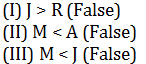# Set-8 Inequalities For SBI PO and SBI Clerk 2019 | Must Go Through These QuestionsDear Aspirants,
We are providing the most important Inequalities for SBI PO 2019, SBI Clerk 2019 and all other competitive bank and insurance exams. These questions have very high chances to be asked in SBI PO 2019, SBI Clerk 2019.
Get the Best Test Series for SBI PO 2019 at the most affordable price (Based on Real Exam Pattern) – Click Here
Download the Best GK Gaming App for Current Affairs and GK (Bank+SSC)– Click here (App No 1)       (App No 2)

Directions:(1-5) In the following question, if all the statements are true. Find the true conclusion among given three conclusion and then further give your answers.
1.2.3.4.5.Directions:(6-9) In these questions, relationship between different elements is shown in the statements. These statements are followed by two conclusions:

6.7.8.9.Check your Answers below:

• Directions:(1-5) In the following question, if all the statements are true. Find the true conclusion among given three conclusion and then further give your answers.
##### 1. QuestionAns:5• ##### 2. Question
In the following question, if all the statements are true. Find the true conclusion among given three conclusion and then further give your answers.Ans:5• ##### 3. Question
In the following question, if all the statements are true. Find the true conclusion among given three conclusion and then further give your answers.Ans:2• ##### 4. Question
In the following question, if all the statements are true. Find the true conclusion among given three conclusion and then further give your answers.Ans:1• ##### 5. Question
In the following question, if all the statements are true. Find the true conclusion among given three conclusion and then further give your answers.Ans:4• ##### 6. Question
In these questions, relationship between different elements is shown in the statements. These statements are followed by twoconclusions. Mark answerAns:4• ##### 7. Question
In these questions, relationship between different elements is shown in the statements. These statements are followed by twoconclusions. Mark answerAns:2• ##### 8. Question
In these questions, relationship between different elements is shown in the statements. These statements are followed by twoconclusions. Mark answerAns:4• ##### 9. Question
In these questions, relationship between different elements is shown in the statements. These statements are followed by twoconclusions. Mark answerAns:5#### About Study Virus

View all posts by Study Virus →

This site uses Akismet to reduce spam. Learn how your comment data is processed.